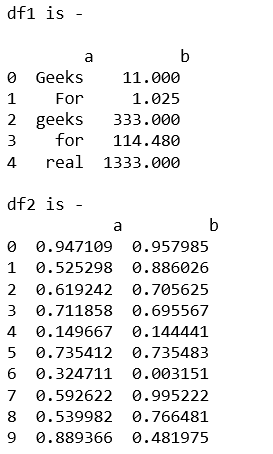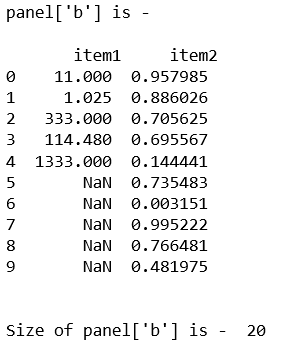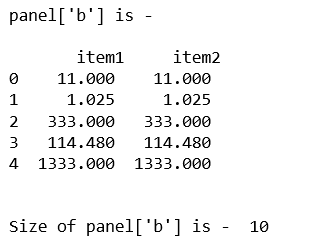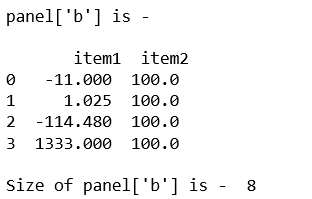Related Articles

# Python | Pandas Panel.size

• Last Updated : 28 Jan, 2019

In Pandas, Panel is a very important container for three-dimensional data. The names for the 3 axes are intended to give some semantic meaning to describing operations involving panel data and, in particular, econometric analysis of panel data.

In Pandas` Panel.size` gives the number of rows if Series. Otherwise return the number of rows times number of columns if DataFrame.

Syntax: Panel.size

Parameters: None

Returns: Returns value in int representing the number of elements in this object.

Code #1:

 `# importing pandas module  ``import` `pandas as pd  ``import` `numpy as np ``   ` `df1 ``=` `pd.DataFrame({``'a'``: [``'Geeks'``, ``'For'``, ``'geeks'``, ``'for'``, ``'real'``],  ``                    ``'b'``: [``11``, ``1.025``, ``333``, ``114.48``, ``1333``]}) ``                     ` `print``(``"df1 is - \n\n"``, df1)`` ` `# Create a 5 * 5 dataframe ``df2 ``=` `pd.DataFrame(np.random.rand(``10``, ``2``), columns ``=``[``'a'``, ``'b'``]) ``print``(``"df2 is - \n\n"``, df2)`Now, let’s create the Panel using dict of df1 and df2, and get the size of that panel.

 `data ``=` `{``'item1'``:df1, ``'item2'``:df2} ``   ` `# creating Panel  ``panel ``=` `pd.Panel.from_dict(data, orient ``=``'minor'``) ``print``(``"panel['b'] is - \n\n"``, panel[``'b'``], ``'\n'``) `` ` ` ` `print``(``"\nSize of panel['b'] is - "``, panel[``'b'``].size) `

Output:Code #2:

 `# importing pandas module  ``import` `pandas as pd  ``import` `numpy as np ``   ` `df1 ``=` `pd.DataFrame({``'a'``: [``'Geeks'``, ``'For'``, ``'geeks'``, ``'for'``, ``'real'``],  ``                    ``'b'``: [``11``, ``1.025``, ``333``, ``114.48``, ``1333``]}) ``                       ` `data ``=` `{``'item1'``:df1, ``'item2'``:df1} ``   ` `# creating Panel  ``panel ``=` `pd.Panel.from_dict(data, orient ``=``'minor'``) ``   ` `print``(``"panel['b'] is - \n\n"``, panel[``'b'``], ``'\n'``) ``   ` `print``(``"\nSize of panel['b'] is - "``, panel[``'b'``].size)   `

Output:Code #3:

 `# importing pandas module ``import` `pandas as pd ``import` `numpy as np `` ` `df1 ``=` `pd.DataFrame({``'a'``: [``'Geeks'``, ``'For'``, ``'geeks'``, ``'real'``], ``                    ``'b'``: [``-``11``, ``+``1.025``, ``-``114.48``, ``1333``]}) `` ` `df2 ``=` `pd.DataFrame({``'a'``: [``'I'``, ``'am'``, ``'dataframe'``, ``'two'``], ``                    ``'b'``: [``100``, ``100``, ``100``, ``100``]}) ``                     ` `data ``=` `{``'item1'``:df1, ``'item2'``:df2}`` ` `# creating Panel ``panel ``=` `pd.Panel.from_dict(data, orient ``=``'minor'``) ``print``(``"panel['b'] is - \n\n"``, panel[``'b'``]) `` ` `print``(``"\nSize of panel['b'] is - "``,  panel[``'b'``].size) `

Output:Attention geek! Strengthen your foundations with the Python Programming Foundation Course and learn the basics.

To begin with, your interview preparations Enhance your Data Structures concepts with the Python DS Course. And to begin with your Machine Learning Journey, join the Machine Learning – Basic Level Course

My Personal Notes arrow_drop_up